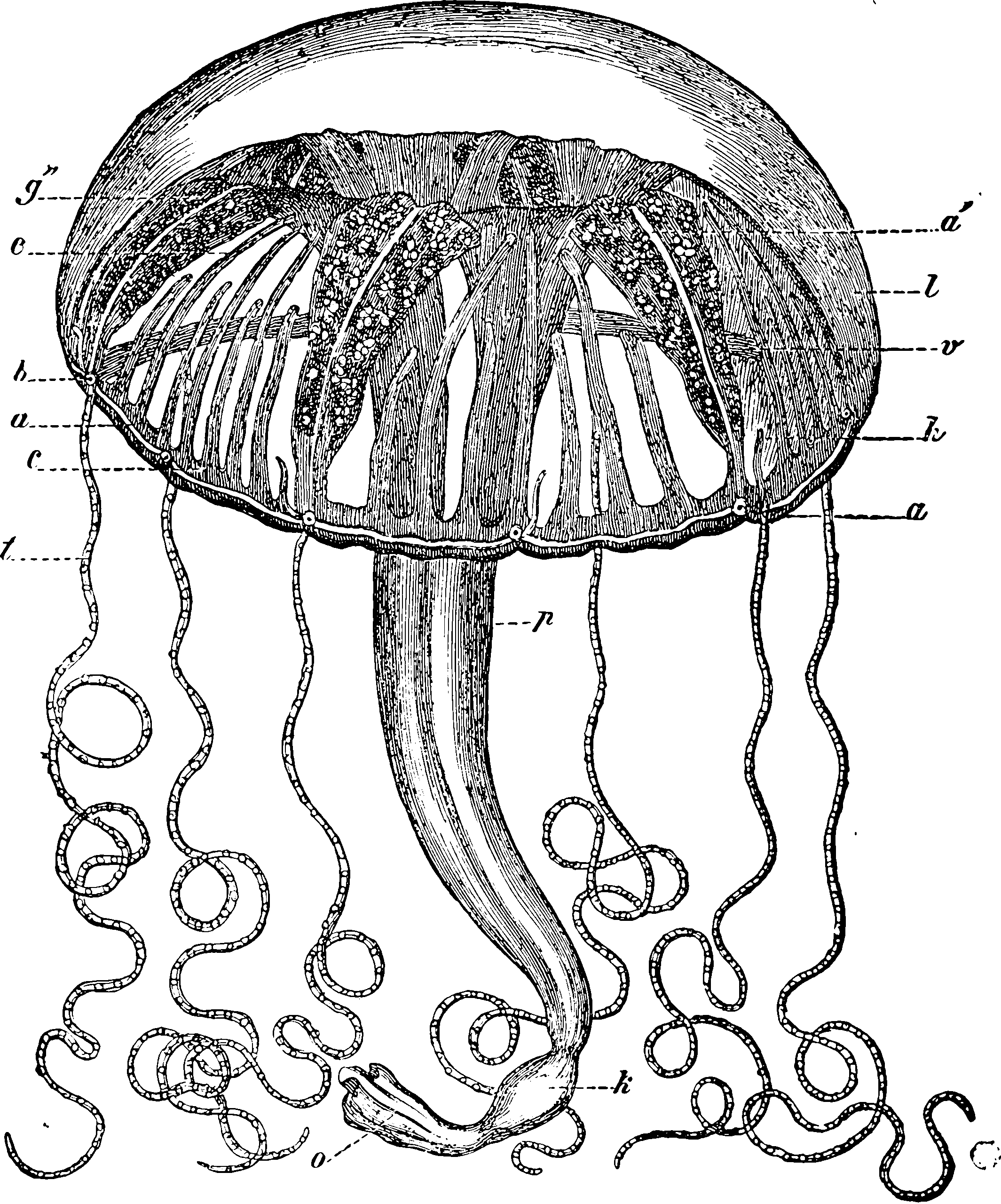# Divisible, decomposable and stable distributions

## Ways of slicing randomness

There are various closely related notions of how to construct random variables as sums of other random variables. I write them out here so that I might finally remember which is which.by ori2uru, CC BY 2.0, via flickr

## Infinitely divisible

The Lévy process quality.

A probability distribution is infinitely divisible if it can be expressed as the probability distribution of the sum of any arbitrary natural number of independent and identically distributed random variables. i.e. The distribution $$F$$ is infinitely divisible if, for every positive integer $$n$$, there exist $$n$$ i.i.d. RVs whose sum

$X_1 + \dots + X_n = S_n \sim F.$

In addition to the classics (Gaussian, Poisson, stable and Gamma processes), Kyprianou (2014) lists various other infinitely divisible ones:

There are many more known examples of infinitely divisible distributions (and hence Lévy processes). Of the many known proofs of infinitely divisibility for specific distributions, most of them are non-trivial, often requiring intimate knowledge of special functions. A brief list of such distributions might include generalised inverse Gaussian (see Good (1953) and Jorgensen (1982)), truncated stable (see Tweedie (1984), Hougaard (1986), Koponen (1995), Boyarchenko and Levendorskii (2002a) and Carr et al. (2003)), generalised hyperbolic (see Halgreen (1979)), Meixner (see Schoutens and Teugels (1998)), Pareto (see Fred W. Steutel (1970), Thorin (1977a)), F-distributions (see Ismail (1979)), Gumbel [see Johnson and Kotz (1970);F. W. Steutel (1973)), Weibull [see Johnson and Kotz (1970);Fred W. Steutel (1970)), lognormal (see Thorin 1977b) and Student t-distribution .

I think the references to Fred W. Steutel (1970), which is an unavailable tech report, can be replaced with refs to Keilson and Steutel (1972) or Fred W. Steutel and van Harn (2003). Most of these are interesting but not actually useful to me since the proofs are often of existence type byt do not give us an easy closed form representation for the component distributions.

Note that infinite divisibility for a given distribution does not necessarily imply that the distribution is divisible in the family we might have assumed — e.g. lognormal RVs are, it turns out, divisible in the family of generalized Γ-convolutions, according to Thorin (1977b), and these generalized Γ-convolutions are closed under addition, although they are not necessarily actual lognormals. Pareto distributions are also included in this class. This large family does sound interesting — see James, Roynette, and Yor (2008). This distinction is made in self decomposability.

Natural exponential families with quadratic variance function have infinite divisibility in all cases except binomial .

## Decomposable

The distribution of $$X$$ is decomposable if there are 2 or more non-constant RVs, not necessarily in the same family, whose sum is equal in distribution. Not a strong property, but the cases where an RV fails to possess even this are curious. 🏗## Self-decomposable

**NB*: This has been updated. AFAICT, decomposable, but the components must be in the same family. Carr et al. (2007) define it this

The distribution of a random variable $$X$$ is said to be self decomposable (Sato 1999 Definition 15.1) if for any constant $$c, 0<c<1$$ there exists an independent random variable say, $$X^{(c)}$$ such that $X \stackrel{\text { law }}{=} c X+X^{(c)}$

While this looks more general than divisible distributions, they note that it self-decomposable distributions form sub-class of infinitely divisible distributions.

## Stable

A distribution or a random variable is said to be stable if a linear combination of two independent copies of a random sample has the same distribution, up to location and scale parameters. (i.e. a stronger property than the infinitely divisible property, which allows other parameters than location and scale.)

This induces (at least?) 2 families of distributions, the discrete and continuous stable families, depending on whether they are supported on the reals or the integers. Presumably other fields get other families. See .

For continuous-valued continuous/discrete indexed stochastic process, $$X(t)$$, $$\alpha$$-stability implies that the marginal law of the value of the process at certain times satisfies a stability equation: $X(a) \simeq W^{1/\alpha}X(b),$ for $$0 < a < b$$, $$\alpha> 0$$ and $$W\sim \operatorname{Unif}([0,1])\perp X$$.

The marginal distributions of such processes are those of the $$\alpha$$-stable processes. For $$\alpha=2$$ we have Gaussians and for $$\alpha=1$$, the Cauchy law.

## Induced processes

### Lévy

All the divisible distributions induce an obvious process - the decomposition of the process into independent increment Markov variates; which is to say, each divisible family induces an associated Lévy process. Classic example: the Gaussian distribution induces a Gauss Markov (i.e. Wiener) process, and the Gamma distribution a Gamma-Lévy process etc.

### Ornstein–Uhlenbeck

This needs a notebook.

### Linear differential

See Lévy SDEs.

🏗 Everything so far has been about sums of RVs; but presumably we could construct similar analyses of other algebraic structures of distributions, e.g. maximum processes. TBD.

## Generalized Gamma Convolutions

The Generalized Gamma Convolutions (GGC) is a construction Barndorff-Nielsen, Maejima, and Sato (2006) that represents some startling (to me) processes using generalized Gamma distributions, including Pareto and Lognormal ones.

This is related to the idea of subordinator convolutions, which convolve a subordinator process with some kernel. The GGC convolves a Gamma distribution with some measure and makes a new divisible distribution.

James, Roynette, and Yor (2008):

we say that a positive r.v. $$\Gamma$$ is a generalized gamma convolution $$(\mathrm{GGC})$$ - without translation term - if there exists a positive Radon measure $$\mu$$ on $$] 0, \infty[$$ such that: \begin{aligned} E\left[e^{-\lambda \Gamma}\right]=& \exp \left\{-\int_{0}^{\infty}\left(1-e^{-\lambda x}\right) \frac{d x}{x} \int_{0}^{\infty} e^{-x z} \mu(d z)\right\} \\ =& \exp \left\{-\int_{0}^{\infty} \log \left(1+\frac{\lambda}{z}\right) \mu(d z)\right\} \\ \text { with: } & \int_{] 0,1]}|\log x| \mu(d x)<\infty \text { and } \int_{[1, \infty[} \frac{\mu(d x)}{x}<\infty \end{aligned}

Pérez-Abreu and Stelzer (2014) generalizes the GGC to vector- and matrix-valued distributions.

### No comments yet. Why not leave one?

GitHub-flavored Markdown & a sane subset of HTML is supported.